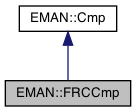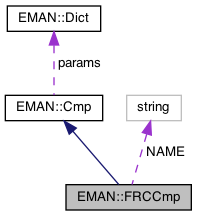EMAN2
EMAN::FRCCmp Class Reference

FRCCmp returns a quality factor based on FRC between images. More...

`#include <cmp.h>`

Inheritance diagram for EMAN::FRCCmp:[legend]
Collaboration diagram for EMAN::FRCCmp:[legend]

## Public Member Functions

float cmp (EMData *image, EMData *with) const
To compare 'image' with another image passed in through its parameters. More...

string get_name () const
Get the Cmp's name. More...

string get_desc () const

TypeDict get_param_types () const
Get Cmp parameter information in a dictionary. More...Public Member Functions inherited from EMAN::Cmp
virtual ~Cmp ()

virtual Dict get_params () const
Get the Cmp parameters in a key/value dictionary. More...

virtual void set_params (const Dict &new_params)
Set the Cmp parameters using a key/value dictionary. More...

static CmpNEW ()

## Static Public Attributes

static const string NAME = "frc"Protected Member Functions inherited from EMAN::Cmp
void validate_input_args (const EMData *image, const EMData *with) constProtected Attributes inherited from EMAN::Cmp
Dict params

## Detailed Description

FRCCmp returns a quality factor based on FRC between images.

Fourier ring correlation (FRC) is a measure of statistical dependency between two averages, computed by comparison of rings in Fourier space. 1 means prefect agreement. 0 means no correlation.

Definition at line 681 of file cmp.h.

## ◆ cmp()

 float FRCCmp::cmp ( EMData * image, EMData * with ) const
virtual

To compare 'image' with another image passed in through its parameters.

An optional transformation may be used to transform the 2 images.

Parameters
 image The first image to be compared. with The second image to be comppared.
Returns
The comparison result. Smaller better by default

Implements EMAN::Cmp.

Definition at line 1417 of file cmp.cpp.

1418{
1419 ENTERFUNC;
1420 validate_input_args(image, with);
1421
1422 int snrweight = params.set_default("snrweight", 0);
1423 int ampweight = params.set_default("ampweight", 0);
1424 int sweight = params.set_default("sweight", 1);
1425 int nweight = params.set_default("nweight", 0);
1427 float minres = params.set_default("minres",200.0f);
1428 float maxres = params.set_default("maxres",8.0f);
1429
1430 vector < float >fsc;
1431 bool use_cpu = true;
1432
1433 if (use_cpu) {
1435 image=image->copy();
1436 with=with->copy();
1437
1438 int sz=image->get_xsize()*image->get_ysize()*image->get_zsize();
1439 float *d1=image->get_data();
1440 float *d2=with->get_data();
1441
1442 for (int i=0; i<sz; i++) {
1443 if (d1[i]==0.0 || d2[i]==0.0) { d1[i]=0.0; d2[i]=0.0; }
1444 }
1445
1446 image->update();
1447 with->update();
1448 image->do_fft_inplace();
1449 with->do_fft_inplace();
1450 image->set_attr("free_me",1);
1451 with->set_attr("free_me",1);
1452 }
1453
1454
1455 if (!image->is_complex()) {
1456 image=image->do_fft();
1457 image->set_attr("free_me",1);
1458 }
1459 if (!with->is_complex()) {
1460 with=with->do_fft();
1461 with->set_attr("free_me",1);
1462 }
1463
1464 fsc = image->calc_fourier_shell_correlation(with,1);
1465 }
1466
1467 int ny = image->get_ysize();
1468 int ny2=ny/2+1;
1469
1470 // The fast hypot here was supposed to speed things up. Little effect
1471// if (image->get_zsize()>1) fsc = image->calc_fourier_shell_correlation(with,1);
1472// else {
1473// double *sxy = (double *)malloc(ny2*sizeof(double)*4);
1474// double *sxx = sxy+ny2;
1475// double *syy = sxy+2*ny2;
1476// double *norm= sxy+3*ny2;
1477//
1478// float *df1=image->get_data();
1479// float *df2=with->get_data();
1480// int nx2=image->get_xsize();
1481//
1482// for (int y=-ny/2; y<ny/2; y++) {
1483// for (int x=0; x<nx2/2; x++) {
1484// if (x==0 && y<0) continue; // skip Friedel pair
1485// short r=Util::hypot_fast_int(x,y);
1486// if (r>ny2-1) continue;
1487// int l=x*2+(y<0?ny+y:y)*nx2;
1488// sxy[r]+=df1[l]*df2[l]+df1[l+1]*df2[l+1];
1489// sxx[r]+=df1[l]*df1[l];
1490// syy[r]+=df2[l]*df2[l];
1491// norm[r]+=1.0;
1492// }
1493// }
1494// fsc.resize(ny2*3);
1495// for (int r=0; r<ny2; r++) {
1496// fsc[r]=r*0.5/ny2;
1497// fsc[ny2+r]=sxy[r]/(sqrt(sxx[r])*sqrt(syy[r]));
1498// fsc[ny2*2+r]=norm[r];
1499// }
1500// free(sxy);
1501// }
1502
1503 vector<float> snr;
1504 if (snrweight) {
1505 Ctf *ctf = NULL;
1506 if (!image->has_attr("ctf")) {
1507 if (!with->has_attr("ctf")) throw InvalidCallException("SNR weight with no CTF parameters");
1508 ctf=with->get_attr("ctf");
1509 }
1510 else ctf=image->get_attr("ctf");
1511
1512 float ds=1.0f/(ctf->apix*ny);
1513 snr=ctf->compute_1d(ny,ds,Ctf::CTF_SNR);
1514 for (int i=0; i<snr.size(); i++) {
1515 if (snr[i]<=0) snr[i]=0.001; // make sure that points don't get completely excluded due to SNR estimation issues, or worse, contribute with a negative weight
1516 }
1517 if(ctf) {delete ctf; ctf=0;}
1518 }
1519
1520 vector<float> amp;
1522
1523 // Min/max modifications to weighting
1524 float pmin = params.set_default("pmin",0);
1525 float pmax = params.set_default("pmax", 0);
1526
1527 if (pmin==0 && minres>0)
1528 pmin=((float)image->get_attr("apix_x")*image->get_ysize())/minres; //cutoff in pixels, assume square
1529
1530 if (pmax==0 && maxres>0)
1531 pmax=((float)image->get_attr("apix_x")*image->get_ysize())/maxres;
1532
1533
1534 double sum=0.0, norm=0.0;
1535
1536 for (int i=0; i<ny/2; i++) {
1537 double weight=1.0;
1538 if (sweight) weight*=fsc[(ny2)*2+i];
1539 if (ampweight) weight*=amp[i];
1540 if (snrweight) weight*=snr[i];
1541// if (snrweight) {
1542// if (snr[i]>0) weight*=sqrt(snr[i]);
1543// else weight=0;
1544// }
1545//if(snr[i]<0) printf("snr[%d] = %1.5g\n",i,snr[i]);
1546 if (pmin>0) weight*=(tanh(5.0*(i-pmin)/pmin)+1.0)/2.0;
1547 if (pmax>0) weight*=(1.0-tanh(i-pmax))/2.0;
1548
1549 sum+=weight*fsc[ny2+i];
1550 norm+=weight;
1551// printf("%d\t%f\t%f\n",i,weight,fsc[ny/2+1+i]);
1552 }
1553
1554 // This performs a weighting that tries to normalize FRC by correcting from the number of particles represented by the average
1555 sum/=norm;
1556 if (nweight && with->get_attr_default("ptcl_repr",0) && sum>=0 && sum<1.0) {
1557 sum=sum/(1.0-sum); // convert to SNR
1558 sum/=(float)with->get_attr_default("ptcl_repr",0); // divide by ptcl represented
1559 sum=sum/(1.0+sum); // convert back to correlation
1560 }
1561
1562 if (image->has_attr("free_me")) delete image;
1563 if (with->has_attr("free_me")) delete with;
1564
1565 EXITFUNC;
1566
1567 if (!Util::goodf(&sum)) sum=-2.0; // normally should be >-1.0
1568
1569 //.Note the negative! This is because EMAN2 follows the convention that
1570 // smaller return values from comparitors indicate higher similarity -
1571 // this enables comparitors to be used in a generic fashion.
1572 return (float)-sum;
1573}
void validate_input_args(const EMData *image, const EMData *with) const
Definition: cmp.cpp:81
Dict params
Definition: cmp.h:132
Ctf is the base class for all CTF model.
Definition: ctf.h:60
float apix
Definition: ctf.h:88
virtual vector< float > compute_1d(int size, float ds, CtfType t, XYData *struct_factor=0)=0
@ CTF_SNR
Definition: ctf.h:68
type set_default(const string &key, type val)
Default setting behavior This can be achieved using a template - d.woolford Jan 2008 (before there wa...
Definition: emobject.h:569
vector< float > calc_radial_dist(int n, float x0, float dx, int inten)
Definition: emdata.cpp:2781
static int goodf(const float *p_f)
Check whether a number is a good float.
Definition: util.h:1112
#define InvalidCallException(desc)
Definition: exception.h:348
#define ENTERFUNC
Definition: log.h:48
#define EXITFUNC
Definition: log.h:49

## ◆ get_desc()

 string EMAN::FRCCmp::get_desc ( ) const
inlinevirtual

Implements EMAN::Cmp.

Definition at line 691 of file cmp.h.

692 {
693 return "Computes the mean Fourier Ring Correlation between the image and reference (with optional weighting factors).";
694 }

## ◆ get_name()

 string EMAN::FRCCmp::get_name ( ) const
inlinevirtual

Get the Cmp's name.

Each Cmp is identified by a unique name.

Returns
The Cmp's name.

Implements EMAN::Cmp.

Definition at line 686 of file cmp.h.

687 {
688 return NAME;
689 }
static const string NAME
Definition: cmp.h:716

References NAME.

## ◆ get_param_types()

 TypeDict EMAN::FRCCmp::get_param_types ( ) const
inlinevirtual

Get Cmp parameter information in a dictionary.

Each parameter has one record in the dictionary. Each record contains its name, data-type, and description.

Returns
A dictionary containing the parameter info.

Implements EMAN::Cmp.

Definition at line 701 of file cmp.h.

702 {
703 TypeDict d;
704 d.put("snrweight", EMObject::INT, "If set, the SNR of 'this' will be used to weight the result. If 'this' lacks CTF info, it will check 'with'. (default=0)");
705 d.put("ampweight", EMObject::INT, "If set, the amplitude of 'this' will be used to weight the result (default=0)");
706 d.put("sweight", EMObject::INT, "If set, weight the (1-D) average by the number of pixels in each ring (default=1)");
707 d.put("nweight", EMObject::INT, "Downweight similarity based on number of particles in reference (default=0)");
708 d.put("zeromask", EMObject::INT, "Treat regions in either image that are zero as a mask (default=0)");
709 d.put("minres", EMObject::FLOAT, "Lowest resolution to use in comparison (soft cutoff). Requires accurate A/pix in image. <0 disables. Default=500");
710 d.put("maxres", EMObject::FLOAT, "Highest resolution to use in comparison (soft cutoff). Requires accurate A/pix in image. <0 disables. Default=10");
711 d.put("pmin", EMObject::FLOAT, "The minimum resolution in pixels.");
712 d.put("pmax", EMObject::FLOAT, "The maximum resolution in pixels.");
713 return d;
714 }

References EMAN::EMObject::FLOAT, EMAN::EMObject::INT, and EMAN::TypeDict::put().

## ◆ NEW()

 static Cmp * EMAN::FRCCmp::NEW ( )
inlinestatic

Definition at line 696 of file cmp.h.

697 {
698 return new FRCCmp();
699 }

## ◆ NAME

 const string FRCCmp::NAME = "frc"
static

Definition at line 716 of file cmp.h.

Referenced by get_name().

The documentation for this class was generated from the following files: